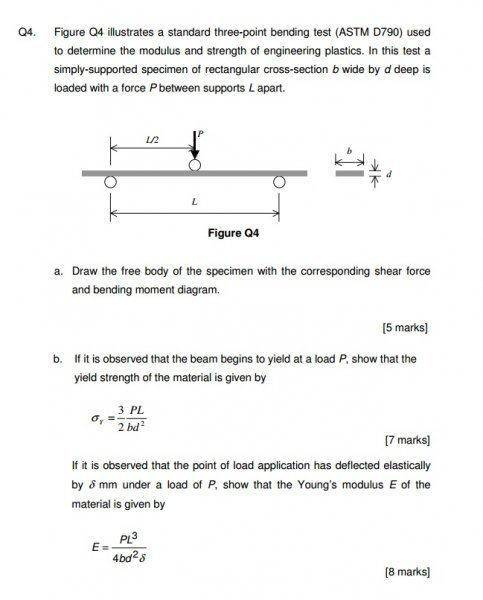# Need help with a mechanics question

Hello

I've attached the question as a JPEG, and I need some help with part b. I've completed part A and got the results:

at x=0: Q= 0 & P/2, M = 0
at x=L/2: Q = P/2 & -P/2, M = PL/4
at x=L: Q = -P/2 & 0, M = 0Any help will be appreciated. Thanks!

Edit: Had to edit so my results from A were more readable. Sorry.

rock.freak667
Homework Helper
Your diagram is correct from what I remember it should look like.

For the second part, the bending moment diagram you drew would give you the maximum bending moment. How would you find the second moment of area I for the cross-section and how do the bending moment and the second moment of area relate to the bending stress?

If the material begins to yield, then your bending stress is equal to what strength?